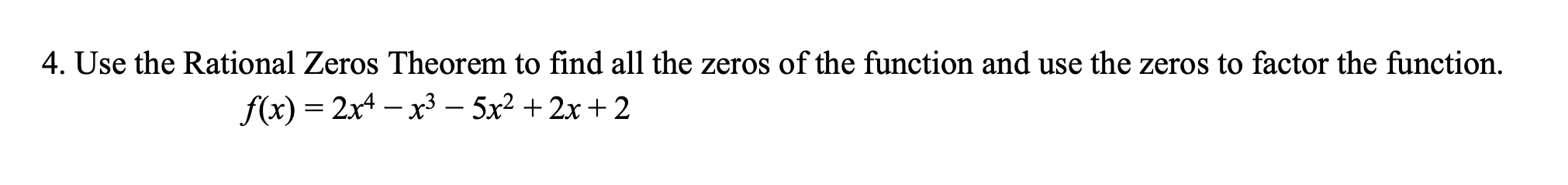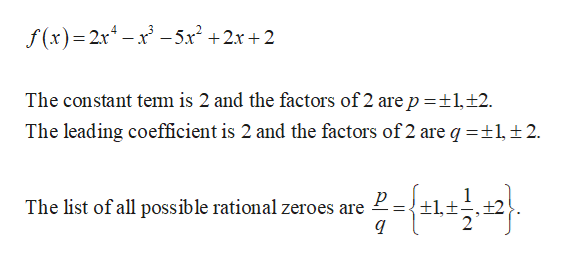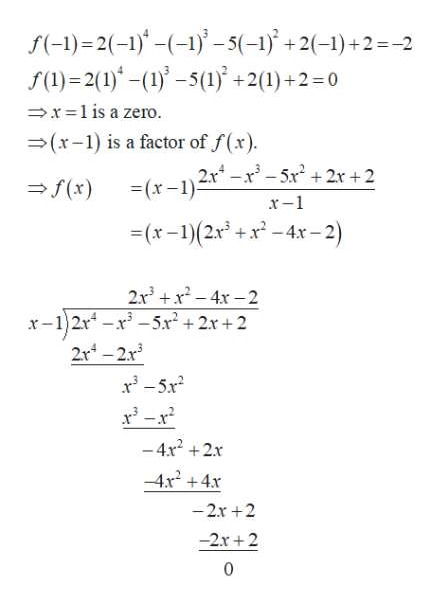# 4. Use the Rational Zeros Theorem to find all the zeros of the function and use the zeros to factor the functionf(x) 2x4-x3 - 5x2 2x+ 2

Question
1 views

4help_outlineImage Transcriptionclose4. Use the Rational Zeros Theorem to find all the zeros of the function and use the zeros to factor the function f(x) 2x4-x3 - 5x2 2x+ 2 fullscreen
check_circle

Step 1

According to the rational roots theorem, if  f(x) is a polynomial with integer coefficients, then every zero of the polynomial has the form p/q where p is a factor of the constant term of  f(x) and q is a factor of the leading coefficient.

Obtain all the possible rational zeroes as follows.help_outlineImage Transcriptionclosef(x) 2x-x-5r2 +2x+2 The constant tem is 2 and the factors of 2 are p 1,+2 The leading coefficient is 2 and the factors of 2 are q =12 р The list of all possible rational zeroes are +1,t,+2 fullscreen
Step 2

Determine which of the possible zeros are actual zeros by substituting these va...help_outlineImage Transcriptionclosef(-1)= 2(-)()-5(-1+2(-1)+2=-2 f()2(1)(-5(1+2(1)+2-0 x1 is a zero. (x-1) is a factor of f(x) = (x-1)r-r -5x 2x +2 f(x) x-1 (x-1)(2x+x-4x-2) 2x3x4x-2 x-12r-x-5x+2r+2 2r-213 3-5x -4x2+2x 4x24x -2r +2 -2r+2 fullscreen

### Want to see the full answer?

See Solution

#### Want to see this answer and more?

Solutions are written by subject experts who are available 24/7. Questions are typically answered within 1 hour.*

See Solution
*Response times may vary by subject and question.
Tagged in

### Algebra